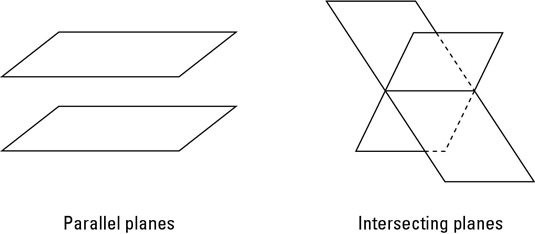##### Geometry Workbook For DummiesWhen two geometric planes interact with each other, it is in one of two ways: as parallel planes or as intersecting planes. Here are the definitions for these two types of relationships between a pair of planes:• Parallel planes: Parallel planes are planes that never cross. The ceiling of a room (assuming it’s flat) and the floor are parallel planes (though true planes extend forever in all directions).

• Intersecting planes: Hold onto your hat — intersecting planes are planes that cross, or intersect. When planes intersect, the place where they cross forms a line. The floor and a wall of a room are intersecting planes, and where the floor meets the wall is the line of intersection of the two planes.